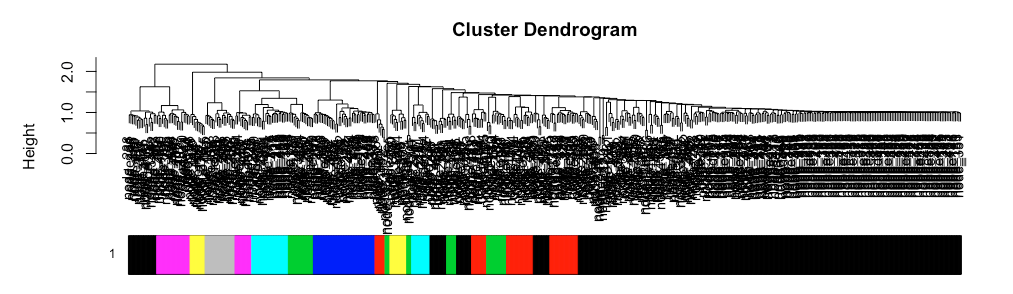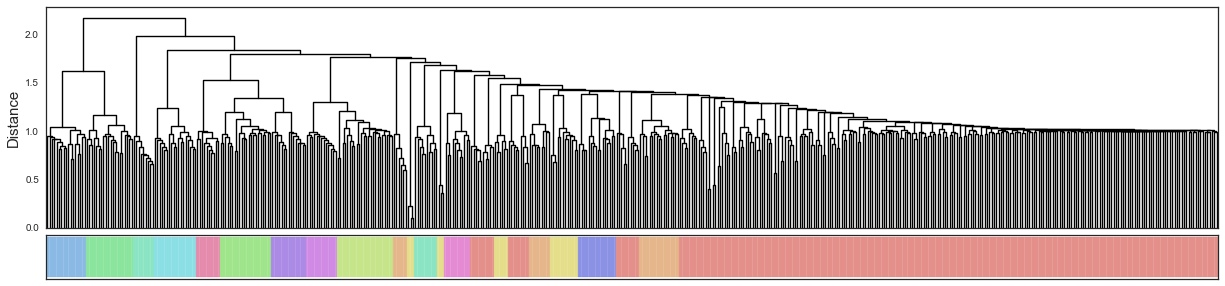Question: dynamicTreeCut and WGCNA show clusters overlapping. Why is this?
1
jol.espinoz20 wrote:

Can somebody explain why I am seeing certain clusters overlap? I tried reproducing the results in Python and in R and I'm seeing a similar pattern.

I have 2 main questions:

(1) Do the colors from WGCNA get repeated? It looks like the 2nd red cluster from the left should be a different color based on my Python plots. Did I plot something incorrectly or is this a known bug of either WGCNA or dynamicTreeCut?

(2) If not (1), why are the clusters overlapping? Is there a parameter I can adjust that controls for this?

Here's my Rcode:

library(dynamicTreeCut)
library(fastcluster)
library(WGCNA)

return(df)
}

# Convert to dissimilarity

Z = hclust(as.dist(df_dism), method="ward.D2")

# Cut the dendrogram
treecut_output = cutreeDynamic(
dendro=Z,
method="hybrid",
distM=df_dism,
minClusterSize = 10,
deepSplit=2,
)

# Plot dendrogram
plotDendroAndColors(
dendro=Z,
colors=treecut_output,
)Here is my python representation using the same parameters: